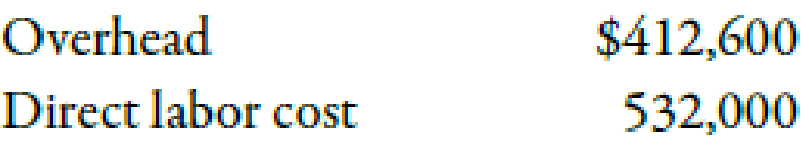# Overhead Variance (Over- or Underapplied), Closing to Cost of Goods Sold At the end of the year, Estes Company provided the following actual information: Estes uses normal costing and applies overhead at the rate of 75% of direct labor cost. At the end of the year, Cost of Goods Sold (before adjusting for any overhead variance) was $1,670,000. Required: 1. Calculate the overhead variance for the year. 2. Dispose of the overhead variance by adjusting Cost of Goods Sold.BuyFind ### Managerial Accounting: The Corners... 7th Edition Maryanne M. Mowen + 2 others Publisher: Cengage Learning ISBN: 9781337115773BuyFind ### Managerial Accounting: The Corners... 7th Edition Maryanne M. Mowen + 2 others Publisher: Cengage Learning ISBN: 9781337115773 #### Solutions Chapter Section Chapter 4, Problem 30BEB Textbook Problem 1545 views ## Overhead Variance (Over- or Underapplied), Closing to Cost of Goods SoldAt the end of the year, Estes Company provided the following actual information:Estes uses normal costing and applies overhead at the rate of 75% of direct labor cost. At the end of the year, Cost of Goods Sold (before adjusting for any overhead variance) was$1,670,000.Required: 1. Calculate the overhead variance for the year. 2. Dispose of the overhead variance by adjusting Cost of Goods Sold.

Expert Solution

1.

To determine

Calculate the overhead variance for the year

### Explanation of Solution

The amount obtained when actual overhead is deducted from applied overhead is known as overhead variance.. Overhead variance is calculated to find whether the overhead is over applied or under applied.

Use the following formula to calculate overhead variance for the year:

Substitute $412,600 for actual overhead and$399,000 for applied overhead in the above formula.

Overheadvariance=$412,600$399,000=$13,600 Therefore, overhead variance - underapplied by$13,600

Expert Solution

2.

To determine